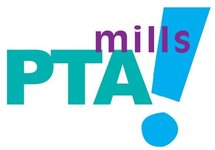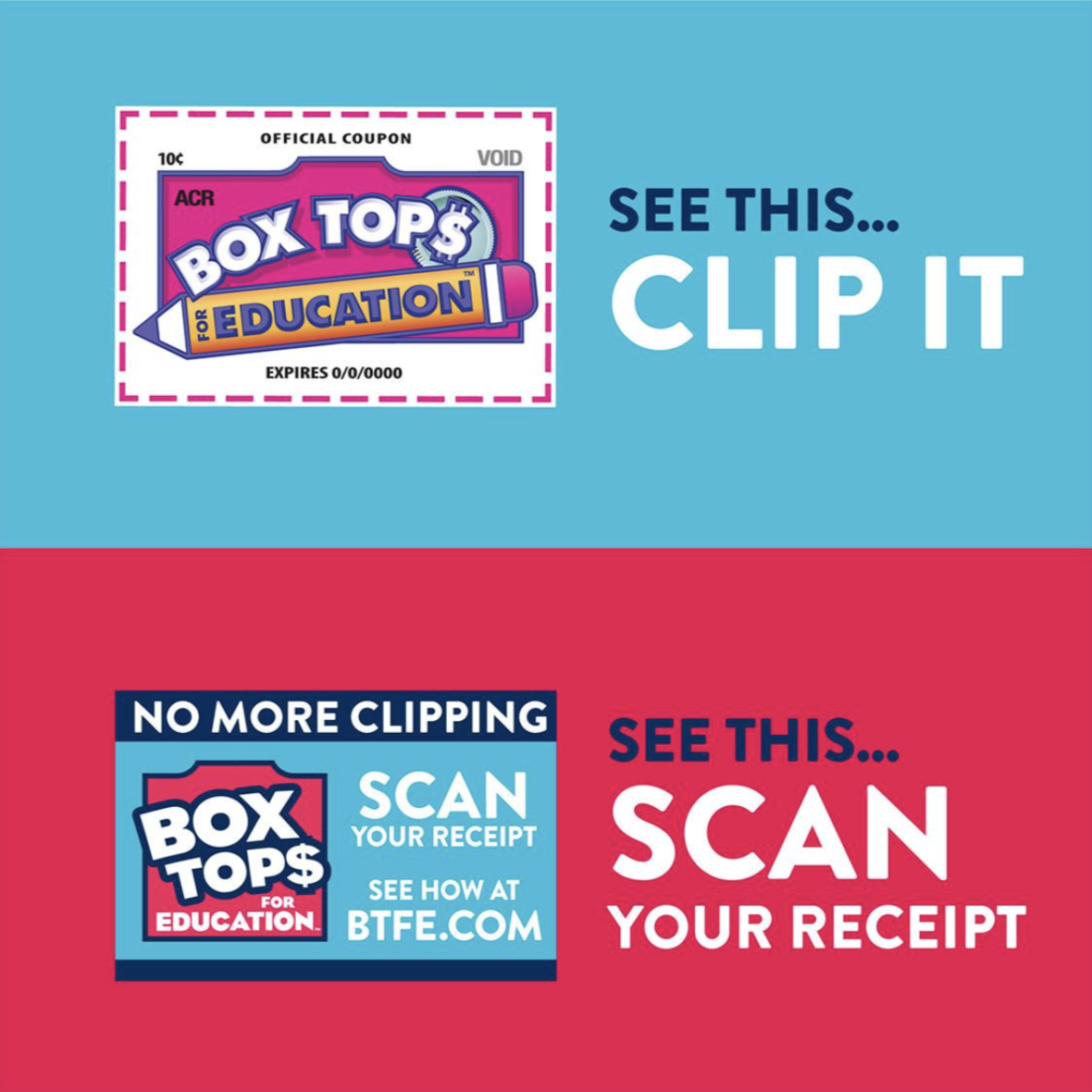## PTA Announcements

 table div table+table+table+table div table{width:100%;padding:0}table div table+table+table+table div table img{width:96.23%;padding:0;float:none}table div table+table+table+table div table td{width:100%;padding:0 1.88% 18px}/* styles */## Box Tops submission deadline: Oct 17Hello Mills Families! It’s Box Top time again! Things are just a little different this year, but I’m hopeful these changes will give us the opportunity to bring in even more FREE MONEY for Mills! Here’s how to participate:

2) Scan your receipt whenever you buy ANY Box Tops participating product. Please note the receipt needs to be scanned within 14 days of purchase, so try to make a habit of scanning right away. If you’re on online shopper, receipts can also be emailed. Go to the app or ​www.boxtops4education.com​ for more information.
3) If your product has the traditional clip-out box tops, collect them to bring to school. Please make sure the expiration date is valid and legible on the clip. Seal them in a baggy and leave them in the pink Box Tops mailbox outside the school office no later than ​Thursday October 17​.

We have a goal to earn at least \$1,000 of free money for Mills through this program. Thank you for helping us get there!

 table div table+table+table+table+table+table+table div table{width:100%;padding:0}table div table+table+table+table+table+table+table div table img{width:96.23%;padding:0;float:none}table div table+table+table+table+table+table+table div table td{width:100%;padding:0 1.88% 18px}/* styles */table div table+table+table+table+table+table+table+table+table+table div table{width:100%;padding:0}table div table+table+table+table+table+table+table+table+table+table div table img{width:96.23%;padding:0;float:none}table div table+table+table+table+table+table+table+table+table+table div table td{width:100%;padding:0 1.88% 18px}/* styles */table div table+table+table+table+table+table+table+table+table+table+table div table td,table.module-10{width:100%;padding:0}table div table+table+table+table+table+table+table+table+table+table+table div table{width:100%;float:none;margin-left:auto;margin-right:auto;padding:0}table div table+table+table+table+table+table+table+table+table+table+table div table a{border:0 none;text-decoration:none}table div table+table+table+table+table+table+table+table+table+table+table div table img{width:100%!important;border:0 none;text-decoration:none}/* styles */
 table div table+table+table+table+table+table+table+table+table+table+table+table div table td,table.module-11{width:100%;padding:0}table div table+table+table+table+table+table+table+table+table+table+table+table div table{width:100%;float:none;margin-left:auto;margin-right:auto;padding:0}table div table+table+table+table+table+table+table+table+table+table+table+table div table a{border:0 none;text-decoration:none}table div table+table+table+table+table+table+table+table+table+table+table+table div table img{width:100%!important;border:0 none;text-decoration:none}/* styles */
 table div table+table+table+table+table+table+table+table+table+table+table+table+table div table td,table.module-12{width:100%;padding:0}table div table+table+table+table+table+table+table+table+table+table+table+table+table div table{width:100%;float:none;margin-left:auto;margin-right:auto;padding:0}table div table+table+table+table+table+table+table+table+table+table+table+table+table div table a{border:0 none;text-decoration:none}table div table+table+table+table+table+table+table+table+table+table+table+table+table div table img{width:100%!important;border:0 none;text-decoration:none}/* styles */
 table div table+table+table+table+table+table+table+table+table+table+table+table+table+table div table{width:100%;padding:0}table div table+table+table+table+table+table+table+table+table+table+table+table+table+table div table img{width:96.23%;padding:0;float:none}table div table+table+table+table+table+table+table+table+table+table+table+table+table+table div table td{width:100%;padding:0 1.88% 18px}/* styles */## SEL Article Study Day

 table div table+table+table+table+table+table+table+table+table+table+table+table+table+table+table+table div table,table.module-15{width:38.49%;float:left;padding:0}table div table+table+table+table+table+table+table+table+table+table+table+table+table+table+table+table div table a{border:0 none;text-decoration:none}table div table+table+table+table+table+table+table+table+table+table+table+table+table+table+table+table div table img{width:100%!important;border:0 none;text-decoration:none}table div table+table+table+table+table+table+table+table+table+table+table+table+table+table+table+table div table td{width:100%;padding:0 20px 20px 0}/* styles */ A few weeks ago, we shared SEL resources for parents and families. As mentioned, we shared there would be an event on Friday, October 25th. Please join an Austin ISD SEL specialist for an article study and discussion on bullying awareness and prevention. There are three available times to attend here at Mills, so be sure to Save the Date and join us for one of the sessions! See the flyer for details.
 table div table+table+table+table+table+table+table+table+table+table+table+table+table+table+table+table+table div table{width:100%;padding:0}table div table+table+table+table+table+table+table+table+table+table+table+table+table+table+table+table+table div table img{width:96.23%;padding:0;float:none}table div table+table+table+table+table+table+table+table+table+table+table+table+table+table+table+table+table div table td{width:100%;padding:0 1.88% 18px}/* styles */## Family Fitness/CATCH Night

 /* styles */ Family Fitness/CATCH (Coordinated Approach To Children's Health) Night is Wednesday, October 16th! Bring your family to the Mills gym to participate in fun fitness activities from 5:00 to 6:30pm. There will be speed and agility exercises, Spikeball, obstacle course relays, pedestrian/bike safety information, health and wellness displays, Fitness gram practice, and more. Family Book fair night will be in the library during the same time. See you there!!!! Mills PE Department
 table div table+table+table+table+table+table+table+table+table+table+table+table+table+table+table+table+table+table+table+table div table{width:100%;padding:0}table div table+table+table+table+table+table+table+table+table+table+table+table+table+table+table+table+table+table+table+table div table img{width:96.23%;padding:0;float:none}table div table+table+table+table+table+table+table+table+table+table+table+table+table+table+table+table+table+table+table+table div table td{width:100%;padding:0 1.88% 18px}/* styles */## Library Book Fair is Coming Soon!

The “Arctic” Fall Book Fair is almost here!

School Book Fair hours:

▪ Oct. 10 and 14-17: 7:30am-4pm
▪ Oct. 18: 7:30am-Noon
▪ Family Night (Oct. 16): 5-8pm
 ▪ Oct. 10 and 14-17: 7:30am-4pm
 ▪ Oct. 18: 7:30am-Noon
 ▪ Family Night (Oct. 16): 5-8pm

Our Fall book fair is coming to the library. We will be previewing a few of the many books available at the fair in the coming weeks and sending home a book fair flyer. As always, books may also be purchased online through Scholastic Book Fairs by accessing this link: https://bookfairs.scholastic.com/bf/mills. Online sales will be available Oct. 10th thru Oct. 23rd, and will be shipped directly to you.

Something new this year is eWallet, a safe, convenient payment option for your little shoppers, and we are urging parents to take advantage of it. Instead of sending cash, you may use a credit card to fund your child’s eWallet account with the convenience of setting a limit. At the conclusion of book fair, any unspent funds are then credited back to you. No currency to worry about!!! If this sounds like something in which you would be interested, follow the website link above. The feature will be available for activating/funding beginning Sept 27th.

We LOVE book fair volunteers, so if you are interested in helping with setting up or taking down the fair or helping cashier and stock during the fair, please sign up on the volunteer link: https://www.signupgenius.com/go/20F084DA5A72AA64-fall. Please note: all AISD school volunteers are now required to complete the Austin Partners In Education (APIE) background check on a yearly basis prior to volunteering at any school event, field trip, or in the classroom. If you haven't completed your APIE application this year, please take a moment to do so now (https://www.austinpartners.org). It will only take about 5 or so minutes of your time to complete the online application, but it takes a few days to process, so please take that into account before planning to volunteer.

 table div table+table+table+table+table+table+table+table+table+table+table+table+table+table+table+table+table+table+table+table+table+table+table div table{width:100%;padding:0}table div table+table+table+table+table+table+table+table+table+table+table+table+table+table+table+table+table+table+table+table+table+table+table div table img{width:96.23%;padding:0;float:none}table div table+table+table+table+table+table+table+table+table+table+table+table+table+table+table+table+table+table+table+table+table+table+table div table td{width:100%;padding:0 1.88% 18px}/* styles */## October Movie Night is Coming!

 table div table+table+table+table+table+table+table+table+table+table+table+table+table+table+table+table+table+table+table+table+table+table+table+table+table div table,table.module-24{width:54.34%;float:right;padding:0}table div table+table+table+table+table+table+table+table+table+table+table+table+table+table+table+table+table+table+table+table+table+table+table+table+table div table a{border:0 none;text-decoration:none}table div table+table+table+table+table+table+table+table+table+table+table+table+table+table+table+table+table+table+table+table+table+table+table+table+table div table img{width:100%!important;border:0 none;text-decoration:none}table div table+table+table+table+table+table+table+table+table+table+table+table+table+table+table+table+table+table+table+table+table+table+table+table+table div table td{width:100%;padding:0 0 20px 20px}/* styles */ Don't Miss October Movie Night featuring Toy Story 4! All are welcome to join in this admission-free, fun family movie night on Friday, October 18th. Families can start arriving at 6:00pm and the movie will begin at 6:30pm. The PTA will sell pizza slices, pepperoni rolls and concessions. RSVP to the event here - https://www.facebook.com/events/506651546561968 Please contact Seychelle McDonald at seychellem43@gmail.com with any questions. See you there!
 table div table+table+table+table+table+table+table+table+table+table+table+table+table+table+table+table+table+table+table+table+table+table+table+table+table+table div table{width:100%;padding:0}table div table+table+table+table+table+table+table+table+table+table+table+table+table+table+table+table+table+table+table+table+table+table+table+table+table+table div table img{width:96.23%;padding:0;float:none}table div table+table+table+table+table+table+table+table+table+table+table+table+table+table+table+table+table+table+table+table+table+table+table+table+table+table div table td{width:100%;padding:0 1.88% 18px}/* styles */## Affiliate News

 table div table+table+table+table+table+table+table+table+table+table+table+table+table+table+table+table+table+table+table+table+table+table+table+table+table+table+table+table div table{width:100%;padding:0}table div table+table+table+table+table+table+table+table+table+table+table+table+table+table+table+table+table+table+table+table+table+table+table+table+table+table+table+table div table img{width:96.23%;padding:0;float:none}table div table+table+table+table+table+table+table+table+table+table+table+table+table+table+table+table+table+table+table+table+table+table+table+table+table+table+table+table div table td{width:100%;padding:0 1.88% 18px}/* styles */## Good News Club

The Good News Club will be on Thursdays starting October 10 through November 21 (six weeks long, excluding Halloween).

3:00-4:00pm. Room TBD.

Snacks are provided. There will be games, songs, Bible lessons and Bible memorization. The club is free. K-5 graders are welcome.

Bring the permission slip to the first meeting on Oct 10th.

See you there!

 table div table+table+table+table+table+table+table+table+table+table+table+table+table+table+table+table+table+table+table+table+table+table+table+table+table+table+table+table+table+table div table{width:100%;padding:0}table div table+table+table+table+table+table+table+table+table+table+table+table+table+table+table+table+table+table+table+table+table+table+table+table+table+table+table+table+table+table div table img{width:96.23%;padding:0;float:none}table div table+table+table+table+table+table+table+table+table+table+table+table+table+table+table+table+table+table+table+table+table+table+table+table+table+table+table+table+table+table div table td{width:100%;padding:0 1.88% 18px}/* styles */## Need to get your message out?

 /* styles */ Submit info to the Mills Messenger. Want to see the Mills Calendar on your Google calendar? Add Mills Calendar to my Google Calendar. Have a friend who isn't getting the Messenger but wants to? Send them this link to sign up: https://madmimi.com/signups/join/7059
 table div table+table+table+table+table+table+table+table+table+table+table+table+table+table+table+table+table+table+table+table+table+table+table+table+table+table+table+table+table+table+table+table+table div table{width:100%;padding:0}table div table+table+table+table+table+table+table+table+table+table+table+table+table+table+table+table+table+table+table+table+table+table+table+table+table+table+table+table+table+table+table+table+table div table img{width:96.23%;padding:0;float:none}table div table+table+table+table+table+table+table+table+table+table+table+table+table+table+table+table+table+table+table+table+table+table+table+table+table+table+table+table+table+table+table+table+table div table td{width:100%;padding:0 1.88% 18px}/* styles */